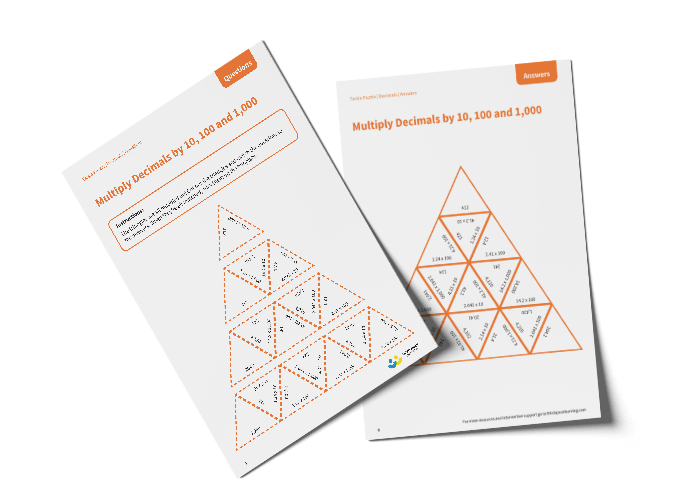# Tarsia Puzzle Multiply Decimals by 10, 100 and 1,000 (Year 5)

The triangles in the tarsia puzzle have all been mixed up. In this multiplying decimals by 10, 100 or 1,000 tarsia puzzle, pupils will need to match the multiplication question to the correct answer to create one large triangle.

This Year 5 multiply decimals by 10, 100 or 1,000 tarsia puzzle worksheet contains:

• One question sheet
• One template sheet

Year 5

Multiplication, Decimals